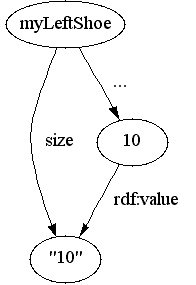# Using XML Schema Datatypes in RDF and DAML+OIL

note: this is no longer (Oct 2002) my favorite design for using XML Schema datatypes in RDF. I now prefer datatype properties, aka Interpretation Properties, and their use in, for example, semantic web travel tools.

Consider this little ontology about a shoe and its size:

```<rdf:RDF
xmlns:rdf="http://www.w3.org/1999/02/22-rdf-syntax-ns#"
xmlns:rdfs="http://www.w3.org/2000/01/rdf-schema#"
xmlns="http://www.daml.org/2000/12/daml+oil#"
xmlns="#">

<rdfs:comment>What I know about shoes</rdfs:comment>
</rdf:Description>

<size>10</size>
<size>10.0</size>
</Shoe>

</rdf:RDF>```

Have I stated a contradiction? Or have I just said that "10" = "10.0"? The latter is, intuitively, closer to what I meant.

This issue, regarding "IEEE floating point numbers, Integers, Boolean values, Dates and Times, etc.", known as c21 in the RDF Schema Working Group, was deferred pending a general solution for primitive datatypes in XML. Now we have such a solution at Candidate Recommendation status: XML Schema Part 2: Datatypes. This document is an attempt to apply that solution to RDF and DAML+OIL.

In that specification, a dataype pairs a set of values with a set of lexical representations. In our example, `10` and `10.0` are lexical representations for the same point in the value space of the decimal datatype.

This notion of lexical representation corresponds neatly to the `value` property from section 2.3 of [RDF@@]:

• we can regard a value whose lexical representation is `10.0` as `[rdf:value "10.0"]`

(sorry; I slipped into notation 3; if you're not familiar, see the primer)

• we can regard the an XML schema datatype (i.e. its value space) as an RDF class, using the URI given in the schema specification: e.g. `http://www.w3.org/2000/10/XMLSchema#decimal` for the decimal datatype(dtnames).

So that the value corresponding to the numeral `10.0` can be written

```<xsd:decimal xmlns:xsd="http://www.w3.org/2000/10/XMLSchema#">
<rdf:value>10.0</rdf:value>
</xsd:decimal>```

or

`[ a xsd:decimal; rdf:value "10.0" ]`

or in diagram form: figure 1 shows the shoe, the decimal number 10 and the numeral 10:Note that in each primitive XML datatype, lexical representations are unambiguous. That is:

```rdf:value util:unambiguousOver xsd:string, xsd:boolean,
xsd:float, xsd:double, xsd:decimal, xsd:timeInstant,
xsd:timeDuration, xsd:recurringInstant, xsd:binary,
xsd:uri.```

where

• unambiugousOver is a class-specific form of daml:Unambiguous; in the style of the DAML+OIL axiomatic semantics, the following is a specification for it:
``` (<=> (PropertyValue ?p unambiguousOver ?c)
(forall (?x ?y ?v)
(=> (and (Type ?x ?c) (Type ?y ?c)
(PropertyValue ?p ?x ?v)
(PropertyValue ?p ?y ?v))
(= ?x ?y)))))```

Then we can introduce a property that relates `size`, whose value is a string, to another property whose value is a decimal:

• valueProperty : Property -> Property
`(forall (?p ?vp ?x ?s)`
`  (=> (and (PropertyValue valueProperty ?p ?vp) (PropertyValue ?vp ?x  ?s)`
`     (exists (?v) (and (PropertyValue ?p ?x ?v) (PropertyValue ?v ?s))) ) )`

A property and a class uniquely determine a value property(keys):

`(forall (?p ?vp1 ?vp2 ?c)`
`  (=> (and (PropertyValue valueProperty ?p ?vp1)`
```           (PropertyValue valueProperty ?p ?vp2)
(PropertyValue rdfs:range ?vp1 ?c)
(PropertyValue rdfs:range ?vp2 ?c)
)
(= ?vp1 ?vp2) ) )```

Using this property, we can state the intended constraint on shoe size as:

```<rdfs:Property about="#size">
<util:valueProperty>
<rdfs:range
rdf:resource="http://www.w3.org/2000/10/XMLSchema#decimal"/>
</util:valueProperty>
</rdfs:Property> ```

so having size `10` and size `10.0` is not a contradiction, but having size `10` and size `11` would be, since 10 and 11 denote different decimals, and sizeDecimal is unique.

These diagrams show the sizeDecimal property related to the size property and the two numerals related to the number and the shoe:figure 2figure 3

That `10` and `11` denote different decimals should be specified in a set of axioms derived from XML Schema Part 2: Datatypes. (yes, that's a lot of work, but it should be very valuable as quality assurance for that spec.) For example, strings are their own values:

```(forall (?s ?v)
(=> (and (PropertyValue rdf:type ?v dt:string)
(PropertyValue rdf:value ?v ?s) )
(= ?v ?s) ) )```

## Using Facets

This section sketches the design, but does not exhaustively enumerate the details.

We can express "decimals greater than 10" by using XML schema facets as properties of classes:

```<rdfs:Class ID="over12">
<!-- over12 is an XMLS datatype based on positiveIntege -->
<!-- with the added restriction that values must be >= 13 -->
<rdfs:subClassOf rdf:resource"#positiveInteger">
<dodt:minInclusive value="13"/>
</xsd:simpleType>```

where postitiveInteger is defined/described ala

```<rdfs:Class rdf:about="#positiveInteger">
<rdfs:subClassOf
rdf:resource"http://www.w3.org/2000/10/XMLSchema#decimal"/>
<dodt:minInclusive>1</dodt:minInclusive>
<dodt:scale>0</dodt:scale>
</rdfs:Class>```

and the dodt: namespace is a translation of the XML Schema datatype facets into DAML+OIL. For example:

```<rdfs:Property about="#minExclusive">
<rdfs:domain resource="...rdfs#Class"/>
<rdfs:comment>axioms:
(forall (?c ?m ?v)
(=> (and (PropertyValue rdf:type ?v ?c)
(PropertyValue minExclusive ?c ?m) )
(> ?v ?m) ) )
</rdfs:comment>
</rdfs:Property>```

## Notes

dtnames
actually, the spec gives the name ...`XMLSchema#xpointer(id("float")` but there's an outstanding request to simplify that to just ...`XMLSchema#float`.
keys
at the December ftf, we talked about an idiom for extending UniqueProperty ala multi-column keys; somebody suggested the design was worked out in Keys for Free in Description Logics.

## References and Related Work

### Related topics

Dan Connolly, Jan 2001
\$Revision: 1.14 \$ of \$Date: 2002/10/21 22:33:51 \$ by \$Author: connolly \$